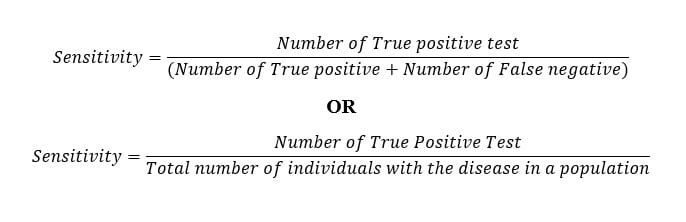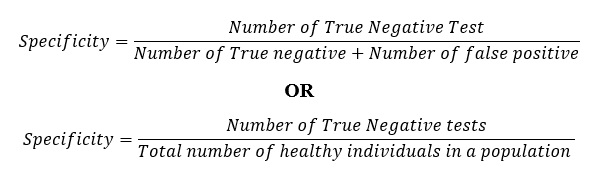# What is Sensitivity, Specificity, False positive, False negative?

Watch Biology Educational Videos

Last Updated on April 18, 2020 by Sagar Aryal

• These are terms that are fundamental to understanding the utility of clinical tests. They are the most essential variables when designing diagnostic tests, in determining how reliable the tests are and also how reliable the results obtained from the results are. When using known samples of a disease, sensitivity and specificity variables can be calculated for evaluation of the test and its results.
• Sensitivity and specificity are independent of the population of interest subject to the tests while Positive predictive value (PPV) and negative predictive value (NPV) is used when considering the value of a test to a clinician and are dependent on the prevalence of the disease in the population of interest.
• The positive predictive value (PPV) is the probability that a subject/sample that returns a positive result really is positive and the negative predictive value (NPV) is the probability that a subject/sample that returns a negative result really is negative
• To evaluate a diagnostic test, it is very important to calculate its sensitivity and specificity in order to determine its effectiveness.
• Positive and Negative terms do not refer to the value of the condition of interest but the presence or absence of the condition. The condition could be a disease, therefore positive means diseased and negative means healthy.## What is Sensitivity?

• Sensitivity is the ability of a test to correctly identify those patients with the disease. It is also known as the True Positive Rate (TPR), i.e. the percentage of sick persons who are correctly identified as having the condition. Therefore sensitivity is the extent to which actual positives are not overlooked.
• For example, a test that correctly identifies all positive samples in a panel is a very sensitive test while a test that only detects 80 % of the true positive samples and 20% of the samples are undetected, hence false negatives in the panel. This test will be termed to have a lower sensitivity because it is missing positives and having a false-negative rate (FPR). This type of error is known as type II errors, false negatives are the failure to reject a false null hypothesis where the sample is negative.
• High sensitivity is very important when detecting a very serious type of infection, for example, the ongoing COVID-19 pandemic, for proper management and treatment. Screening tests should have a very limited type-2 error, if not none for accurate identification of the disease. Theis a screening test for COVID-19 with a 95% sensitivity rate.
• To determine the sensitivity of a test probability (percentage) that a sample tests positive given that the patient has the disease,
• Calculation:## What is Specificity?

• This is the ability of a clinical test to correctly identify those patients without the disease. It is also known as the True Negative Rate (TNR), i.e the percentage of healthy people who are correctly identified as not having the condition. A test that can identify all sample tests from healthy individuals to be negative is very specific.
• Therefore, a test with 100% specificity correctly identifies all patients without the disease, while a test with 80% specificity correctly reports 80% of patients without the disease as test negative (true negatives) but 20% patients without the disease are incorrectly identified as to test positive (false positives).
• A test that has a high sensitivity but low specificity causes many patients who do not have the disease being told of a possibility that they have a disease, subjecting them to further testing. Ideally, the test should be 100% accurate but this is an unrealistic scenario. Alternatively, subjecting patients to a test with high sensitivity and low specificity and a second test with low sensitivity and high specificity can identify all false positives and false negatives.## Sensitivity vs Specificity mnemonic

• SnNouts and SpPins is a mnemonic to help you remember the difference between sensitivity and specificity.
• SnNout: A test with a high sensitivity value (Sn) that, when negative (N), helps to rule out a disease (out).
• SpPin: A test with a high specificity value (Sp) that, when positive (P) helps to rule in a disease (in).

## What is False positive and False negative?

• The true/false refers to the assigned classification being correct or incorrect while positive/negative refers to the assignment to a positive or negative category of results.
• These terminologies are dependent on the population subject to the test. Normally, when there is a disease outbreak, diagnostic tests are done to determine if an individual has the disease or not.
• For persons who are sick, the test outcome is positive while those without the disease, the test outcome will be negative. But in some circumstances, the test results may not match the individual’s status, therefore they can be defined as:
• False-positive: Healthy people incorrectly identified as sick
• False-negative: Sick people incorrectly identified as healthy.
• A true-positive means that the individual who is sick has been correctly identified to have the disease while an individual who is a true-negative, means the individual who does not have the disease has been correctly diagnosed to not having the disease.

## Example of Sensitivity and specificity

Assumption: You have a new rapid diagnostic test being evaluated for the screening of COVID-19, on the specific antibodies produces against the virus, SARS-CoV-2. You have a sample size of 600 people and by validity, there are samples that you know definitely have the disease (480) and/or healthy individual samples from the disease in question (120). After running the test, then you compare the results to their know disease staus and find that:

• True positive (test positive and are correctly positive) = 480
• False-positive (test positive but are actually negative) = 15
• True negative (test negative and are genuinely negative) = 100
• False-negative (test negative but are actually positive) =5

## Tabulated Results

 Positive Negative Total True Positive 480 – 480 False Positive 15 – 15 True Negative – 100 100 False Negative – 5 5 Total 495 105 600
•  Sensitivity = 480/(480+5)= 0.98
• Therefore, the test has a 98% sensitivity.
• Specificity = 100/(100+15)=0.87
• Therefore, the test has 87% specificity.

## Positive Predictive Value (PPV) and Negative Predictive Value (NPV)

• Positive predictive value (PPV) is a probability value that a sample test positive is really positive while the negative predictive value (NPV) is the probability value that a sample test is really negative.
• They are useful indexes in evaluating sample results and they can be calculated like sensitivity and specificity. These values can also be used to calculate sensitivity and specificity.
• Therefore, it is correct to say that Sensitivity and specificity evaluate the test while PPV and NPV evaluate the results.

## Calculation

• Positive Predictive Value (PPV)=(Number of true positive )/(Number of true positives+number of false positives)

OR

• Positive Predictive Value (PPV)=(Number of True positive)/(Total of positive results)

• Negative Predictive Value (NPV)=(Number of True Negative)/(Number of true negative+Number of False-negative)

OR

• Negative Predictive Value=(Number of True Negative)/(Total of negative results)
• Using the table above, we can calculate PPV and NPV as follows:
• PPV=480/(480+15)=0.97….97%
• NPV=100/(100+5)= 0.95…95%
• Therefore, if a test is positive, there is a 97% chance that it is correct and if the result is negative, there is a 95% chance it is correct.
• The opposite values are False Discovery Rate (FPV) for Positive Predictive Value (PPV) and False Omission Rate (FOR) for Negative Predictive Value (NPV).

## References and Sources

1. https://www.ncbi.nlm.nih.gov/pmc/articles/PMC2636062/
3. 9% – https://www.technologynetworks.com/analysis/articles/sensitivity-vs-specificity-318222
6. 2% – https://anesthesia.mcmaster.ca/docs/librariesprovider8/research/methodology/statistical-notes/clinical-tests–sensitivity-and-specificity.pdf?sfvrsn=25424405_4
8. 2% – http://sheepshoot.com/Sensitivity_and_specificity
9. 1% – https://www.ncbi.nlm.nih.gov/pmc/articles/PMC4966236/
10. 1% – https://www.fosters.com/lifestyle/20200312/covid-19-more-tests-and-more-accurate-results-are-needed
11. 1% – https://en.wikipedia.org/wiki/Sensitivity_and_specificity
12. 1% – https://ebn.bmj.com/content/23/1/2
13. 1% – http://www.mas.ncl.ac.uk/~njnsm/medfac/MBBS/handout.pdf